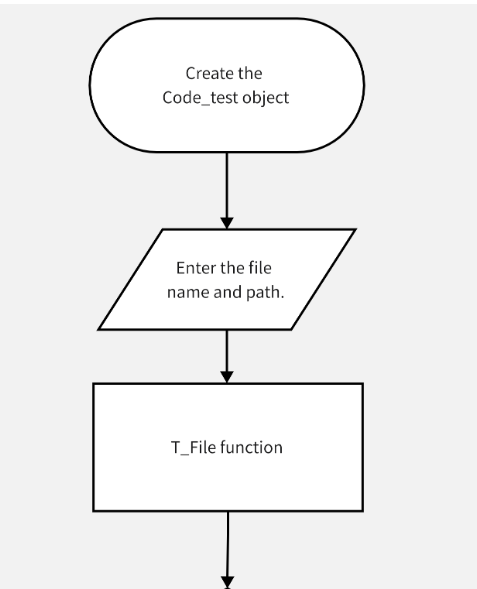# EE308 LAB 1-2

 The Link Your Class bbs.csdn.net/forums/MUEE308FZU202201 The Link of Requirement of This Assignment bbs.csdn.net/topics/608734618 The Aim of This Assignment extract keywords of different levels MU STU ID and FZU STU ID 20122934_832001114

PSP Form

Personal Software Process StagesEstimated time(min）Time(min）
Planning3050
Estimate100100
Development1010
Analysis100100
Design Spec--
Design Review1030
Coding Standard3040
Design4050
Coding100100
Code Review9080
Test120130
Test Report6060
Postmortem & Process Improvement Plan· Design Review4050
Summary630700

Procedure

Accroding to the task article, It is obvious that we need to know how to read the file and read the contents of the file. Firstly, we should know how to divide the keywords. the test file only contains the key fields for switch, if-else, and case, so we can finish it quickly.After dividing the structure of these keywords, the number of occurrences is counted.I developed a simple data reading function, can enhance the expansion, all the keywords are written into an array, and then each time from the array of two-dimensional search, find the corresponding keywords, and then count the number. So do that, we can finish the task.

Flow chartAccording to the  c code make a judgment

to find the number fo the factorsThe Code

``````#include <stdio.h>
#include <iostream>
#include <string>
#include <fstream>
#include <cstring>
#include <algorithm>
#include <iostream>
#include <sstream>
#include <stack>
using namespace std;
const int maxn = 100;
string &replace_all(string &str, const string &old_value, const string &new_value)
{
while (true)
{
string::size_type pos(0);
if ((pos = str.find(old_value)) != string::npos)
str.replace(pos, old_value.length(), new_value);
else
break;
}
return str;
}
int main()
{
int total_num = 0;
int switch_num = 0;
int case_num = 0;
int if_else_num = 0;
int if_elseif_else_num = 0;
int level;
stack<int> if_else_container;
string codes;
string filename;
ifstream inFile;

string keyword={"void","char","int","float","double","short","long","signed","unsigned",
"struct","union","enum","typedef","sizeof","auto","static","register","extern","const","volatile",
"return","continue","break","goto","if","else","switch","case","default","for","do","while"};

cout << "Type in the file name: " ;
cin >> filename;
cout << "Type in the requirement 1 2 3 or 4 ";
cin >> level;

inFile.open(filename.c_str());
if(inFile.fail())
{
cout << "\nThe file was not successfully opened."
<< "\nPlease check that the file currently exists or type the error name."
<< endl;
exit(1);
}

//Basic Requirement

string Codes[maxn];
int counter=0;

while (getline(inFile, codes))
{
int i,j;
string a;
for(i=0; i<codes.size(); i++)
{
if(codes[i]>='a' && codes[i]<='z')
{
for(j=i; codes[j]>='a' && codes[j]<='z'; j++);
a=codes.substr(i,j-i);
Codes[counter]=a;
counter++;
for(int k=0;k<32;k++)
{
if(a == keyword[k])
{
total_num++;
break;
}
}
i=j;
}
else
{
j=i;
}
}
if (codes.rfind("else if") < maxn)
{
if_else_container.push(1);
}
else if (codes.rfind("else") < maxn)
{
if_else_container.push(2);
}
else if (codes.rfind("if") < maxn)
{
if_else_container.push(0);
}

int index = 0;

replace_all(codes, "(", " ");
replace_all(codes, ")", " ");
replace_all(codes, "{", " ");
replace_all(codes, ":", " ");
replace_all(codes, ";", " ");
istringstream str(codes);
string filter;
string last = "";
}
stack<int> tmp;
while (!if_else_container.empty())
{
int top_element = if_else_container.top();
if_else_container.pop();
if (top_element == 2)
{
tmp.push(2);
}
if (top_element == 1)
{
tmp.push(1);
}
if (top_element == 0)
{
if (tmp.top() == 2)
{
if_else_num++;
tmp.pop();
}
else if (tmp.top() == 1)
{
if_elseif_else_num++;
while (tmp.top() != 2)
{
tmp.pop();
}
tmp.pop();
}
}
}
cout << "total numbers: " << total_num << endl;

int m,n;
int corresponding_case[maxn] = {0};
for(m=0; m<counter; m++)
{
if(Codes[m] == "switch")
{
switch_num++;
for(int n=m+1; n<counter; n++)
{
if(Codes[n] == "case")
corresponding_case[switch_num]++;
else if(Codes[n] == "switch")
{
m=n-1;
break;
}
}

}
}
if(level >= 2)
{
cout << "switch numbers: " << switch_num << endl;
for(int a=1; a<=switch_num; a++)
{
cout << " the number of case corresponding to switch" << a << ": " << corresponding_case[a] << endl;
}
}

//Uplifting Requirement

if(level >= 3)
{
cout << "if-else numbers: " << if_else_num << endl;
}
if(level >= 4)
{
cout << "if-elseif-else numbers: " << if_elseif_else_num << endl;
}
}``````

The Summary

First of all, this is my first time classification task, the task that I deeply realize that my programming ability not so beautiful, I also have many shortcomings of my analysis of the problem is not clear, this led to I will complicate and difficult to write a simple code, I hope I can continue to grow and improve the level of their code.

Secondly, this experiment introduced me to GitHub for the first time. Although it was difficult for me to access Github for some special reasons, I finally succeeded in creating my account and successfully storing my code.

Finally, I realized the importance of time management. Using the psp spreadsheet, I knew when to do each task, which gave me a clearer and more specific goal and a sense of urgency. Although there is a certain gap between the completion time and the estimated time, I will continue to improve my code level, and strive to be able to complete the task within the scheduled time.

...全文
86 回复 打赏 收藏 转发到动态 举报RHCA存储管理RH436视频.zipEE308 LAB 12 Course Summary
The Link Your Class EE308 MIEC The Link of Requirement of This Assignment LAB 12 Course Summary The Aim of This Assignment Course Summary MU STU ID and FZU STU ID 19103387_831902225 Summary (1) Compared with the course objectives and expectat...EE308 LAB 11 Software Testing
The Link Your Class EE308 MIEC The Link of Requirement of This Assignment LAB 11 Software Testing The Aim of This Assignment Software Testing MU STU ID and FZU STU ID 19103387_831902225 Preparation Learn related knowledge about Junit Test for...EE308 LAB 10 Alpha Sprint Improvements
The Link Your Class EE308 MIEC The Link of Requirement of This Assignment Lab 10 Alpha Sprint Improvements Group number 7 - A Group Leader’s MU STU ID and FZU STU ID 韦知辰19103387_831902225 Teammate1’s MU STU ID and FZU STU ID 罗天19104642_831902...EE308 LAB 9 UML Exercises
Requirement Please issue your Lab Blog on CSDN. The Link of your blog of this lab and This Lab Sheet (This word file) must be submitted to Moodle, or you will lose the score of this Lab. ( VERY IMPORTANT : You should submit TWO things) Modeling must use p

285发帖与我相关我的任务• 近7日
• 近30日
• 至今